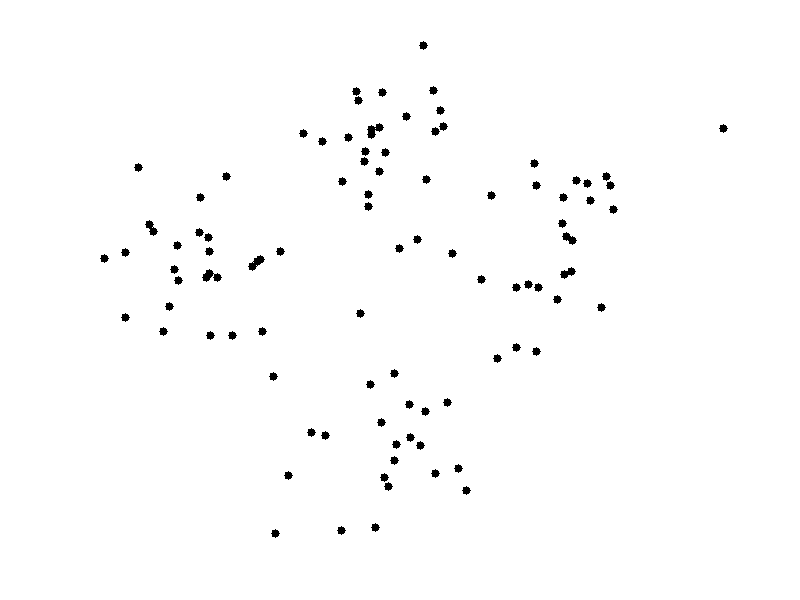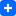# Sorting strings and Overriding std::sort comparison

At that post, I try to illustrate one of the use case of comparison overriding for std::sort on top of a simple problem. Our problem is as follows:

Write a method to sort an array of strings so that all the anagrams are next to each other.

# Project Euler - Problem 13

Here we have another qualified problem from Project Euler. You might want to work out the problem before see my solution.

The basic idea of my solution is to not use all the digits of the given numbers, instead extract the part of the each number that is necessary to sum up to conclude the first 10 digits of the result. I try to explain my approach at the top of the source code with my lacking MATH english. If you have any problem for that part please leave me a comment. Continue reading Project Euler - Problem 13

# Project Euler - Problem 12

As a 2 years researcher, I feel a bit rusty to code.  I search a good set of execises to hone my abilities again and I stumbled upon Project Euler. This site hosts increasing number of very well formed algorithmic problems and discussions. It ranges very basic problems to very high level ones, requiring profound knowledge and practice.

After that intro. I want to introduce one of the example question from Project Euler. NOTE THAT, IF YOU ALREADY KNOW THE SITE AND YOU TRY TO SOLVE THAT PROBLEM, DO NOT CHEAT YOURSELF.

Here is the problem statement we try to solve. Continue reading Project Euler - Problem 12

# Some inefficient algorithms you don't need to know!

Here we have some stupidly clever algorithms that are faster in execution but slower in convergence to solution.

First candidate to introduce you is Bogo Sort. Its idea is simpler. Shuffle the numbers until it finds the correct order. Complexity of the algorithm can be approximated as O(n.n!) (efficient hah ?). Its pseudo code can be written as;

``````while not isInOrder(deck):
shuffle(deck);``````

Next one is known as Stooge Sort. The idea is to sort the initial 2/3 part t Continue reading Some inefficient algorithms you don't need to know!

# Anomaly detection and a simple algorithm with probabilistic approach.

What is anomaly detection? It is the way of detecting a outlier data point among the other points that have a some kind of logical distribution.  Outlier one is also anomalous point (Figure 1)

What are the applications?

•  Fraud user activity detection - it is a way of detecting hacker activities on web applications or network connections by considering varying attributes of the present status. For example , an application can keep track of the user's inputs to website and the work load that he proposes to system. Considering these current attribute values detection system decide a particular fraud action and kick out the user if there is.
• Data center monitoring - You might be governing a data center with vast amount of computers so it is really hard to check each computer regularly for any flaw. A anomaly detection system might be working by considering network connection parameters of the computers, CPU and Memory Loads, it detect any problem on computer. Continue reading Anomaly detection and a simple algorithm with probabilistic approach.

# How K-means clustering works

K-means is the most primitive and easy to use clustering algorithm (also a Machine Learning algorithm).
There are 4 basic steps of K-means:

1. Choose K different initial data points on instance space (as initial centroids) - centroid is the mean points of the clusters that overview the attributes of the classes-.
2. Assign each object to the nearest centroid.
3. After all the object are assigned, recalculate the centroids by taking the averages of the current classes (clusters)
4. Do 2-3 until centroid are stabilized.

Caveats for K-means:

• Although it can be proved that the procedure will always terminate, the k-means algorithm does not necessarily find the most optimal configuration, corresponding to the global objective function minimum.
• The algorithm is also significantly sensitive to the initial randomly selected cluster centres. The k-means algorithm can be run multiple times to reduce this effect.

Here is the basic animation to show the intuition of K-means.# A solution for a Greedy Choice Algorithm Solution from TopCoder

``````
/**
* You are playing a computer game and a big fight is planned between two armies.
* You and your computer opponent will line up your respective units in two rows,
* with each of your units facing exactly one of your opponent's units and vice versa.
* Then, each pair of units, who face each other will fight and the stronger one will be
* victorious, while the weaker one will be captured. If two opposing units are equally strong,
* your unit will lose and be captured. You know how the computer will arrange its units,
* and must decide how to line up yours. You want to maximize the sum of the strengths of
* your units that are not captured during the battle.
* You will be given a int[] you and a int[] computer that specify the strengths of
* the units that you and the computer have, respectively. The return value should be an int,
* the maximum total strength of your units that are not captured.
*
* FROM TOP CODER
*
* {651, 321, 106, 503, 227, 290, 915, 549, 660, 115,
* 491, 378, 495, 789, 507, 381, 685, 530, 603, 394,
* 7, 704, 101, 620, 859, 490, 744, 495, 379, 781,
* 550, 356, 950, 628, 177, 373, 132, 740, 946, 609,
* 29, 329, 57, 636, 132, 843, 860, 594, 718, 849}
*
* computer array
* {16, 127, 704, 614, 218, 67, 169, 621, 340, 319,
* 366, 658, 798, 803, 524, 608, 794, 896, 145, 627,
* 401, 253, 137, 851, 67, 426, 571, 302, 546, 225,
* 311, 111, 804, 135, 284, 784, 890, 786, 740, 612,
* 360, 852, 228, 859, 229, 249, 540, 979, 55, 82}
*
* Returns: 25084
*
*/

import java.util.Arrays;
public class Main {

public void findMaxStrength(int[] computer, int[] player){
Arrays.sort(computer);
Arrays.sort(player);
int index = computer.length-1;
int smallIndex = 0;
for(int i = computer.length-1; i>=0; i--){
if(player[index] <= computer[i]){
shift(player, smallIndex, index);
index--;
}else{
index--;
}
}
}

public int[] shift(int[] a, int index1, int index2){
int e = a[index1];
for(int i = index1; i``````
``````
``` algorithmgreedyJavatop_coder```
``` ```